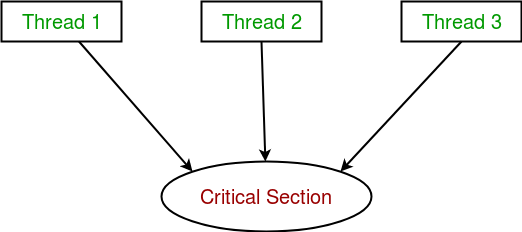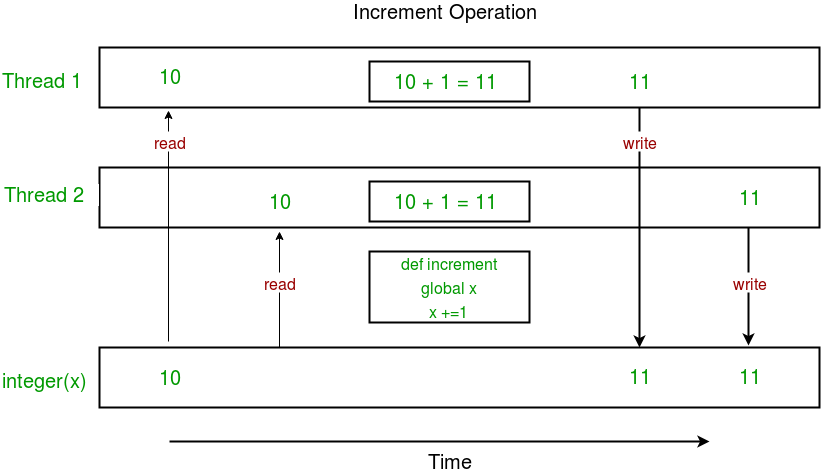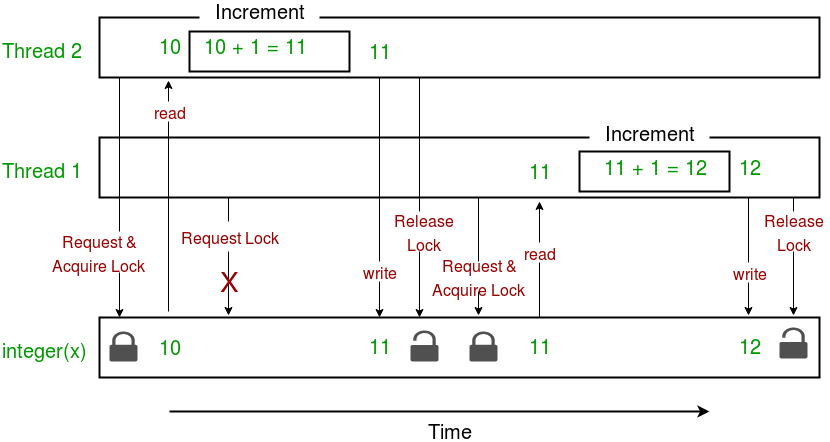Open in App
Not now

## Related Articles

• Write an Interview Experience

# Multithreading in Python | Set 2 (Synchronization)

• Difficulty Level : Medium
• Last Updated : 28 Aug, 2019

Thread synchronization is defined as a mechanism which ensures that two or more concurrent threads do not simultaneously execute some particular program segment known as critical section.

Critical section refers to the parts of the program where the shared resource is accessed.

For example, in the diagram below, 3 threads try to access shared resource or critical section at the same time.Concurrent accesses to shared resource can lead to race condition.

A race condition occurs when two or more threads can access shared data and they try to change it at the same time. As a result, the values of variables may be unpredictable and vary depending on the timings of context switches of the processes.

Consider the program below to understand the concept of race condition:

 `import` `threading`` ` `# global variable x``x ``=` `0`` ` `def` `increment():``    ``"""``    ``function to increment global variable x``    ``"""``    ``global` `x``    ``x ``+``=` `1`` ` `def` `thread_task():``    ``"""``    ``task for thread``    ``calls increment function 100000 times.``    ``"""``    ``for` `_ ``in` `range``(``100000``):``        ``increment()`` ` `def` `main_task():``    ``global` `x``    ``# setting global variable x as 0``    ``x ``=` `0`` ` `    ``# creating threads``    ``t1 ``=` `threading.Thread(target``=``thread_task)``    ``t2 ``=` `threading.Thread(target``=``thread_task)`` ` `    ``# start threads``    ``t1.start()``    ``t2.start()`` ` `    ``# wait until threads finish their job``    ``t1.join()``    ``t2.join()`` ` `if` `__name__ ``=``=` `"__main__"``:``    ``for` `i ``in` `range``(``10``):``        ``main_task()``        ``print``(``"Iteration {0}: x = {1}"``.``format``(i,x))`

Output:

```Iteration 0: x = 175005
Iteration 1: x = 200000
Iteration 2: x = 200000
Iteration 3: x = 169432
Iteration 4: x = 153316
Iteration 5: x = 200000
Iteration 6: x = 167322
Iteration 7: x = 200000
Iteration 8: x = 169917
Iteration 9: x = 153589```

In above program:

• Two threads t1 and t2 are created in main_task function and global variable x is set to 0.
• increment function will increment the global variable x by 1 in each call.

The expected final value of x is 200000 but what we get in 10 iterations of main_task function is some different values.

This happens due to concurrent access of threads to the shared variable x. This unpredictability in value of x is nothing but race condition.

Given below is a diagram which shows how can race condition occur in above program:Notice that expected value of x in above diagram is 12 but due to race condition, it turns out to be 11!

Hence, we need a tool for proper synchronization between multiple threads.

Using Locks

threading module provides a Lock class to deal with the race conditions. Lock is implemented using a Semaphore object provided by the Operating System.

A semaphore is a synchronization object that controls access by multiple processes/threads to a common resource in a parallel programming environment. It is simply a value in a designated place in operating system (or kernel) storage that each process/thread can check and then change. Depending on the value that is found, the process/thread can use the resource or will find that it is already in use and must wait for some period before trying again. Semaphores can be binary (0 or 1) or can have additional values. Typically, a process/thread using semaphores checks the value and then, if it using the resource, changes the value to reflect this so that subsequent semaphore users will know to wait.

Lock class provides following methods:

• acquire([blocking]) : To acquire a lock. A lock can be blocking or non-blocking.
• When invoked with the blocking argument set to True (the default), thread execution is blocked until the lock is unlocked, then lock is set to locked and return True.
• When invoked with the blocking argument set to False, thread execution is not blocked. If lock is unlocked, then set it to locked and return True else return False immediately.
• release() : To release a lock.
• When the lock is locked, reset it to unlocked, and return. If any other threads are blocked waiting for the lock to become unlocked, allow exactly one of them to proceed.

Consider the example given below:

 `import` `threading`` ` `# global variable x``x ``=` `0`` ` `def` `increment():``    ``"""``    ``function to increment global variable x``    ``"""``    ``global` `x``    ``x ``+``=` `1`` ` `def` `thread_task(lock):``    ``"""``    ``task for thread``    ``calls increment function 100000 times.``    ``"""``    ``for` `_ ``in` `range``(``100000``):``        ``lock.acquire()``        ``increment()``        ``lock.release()`` ` `def` `main_task():``    ``global` `x``    ``# setting global variable x as 0``    ``x ``=` `0`` ` `    ``# creating a lock``    ``lock ``=` `threading.Lock()`` ` `    ``# creating threads``    ``t1 ``=` `threading.Thread(target``=``thread_task, args``=``(lock,))``    ``t2 ``=` `threading.Thread(target``=``thread_task, args``=``(lock,))`` ` `    ``# start threads``    ``t1.start()``    ``t2.start()`` ` `    ``# wait until threads finish their job``    ``t1.join()``    ``t2.join()`` ` `if` `__name__ ``=``=` `"__main__"``:``    ``for` `i ``in` `range``(``10``):``        ``main_task()``        ``print``(``"Iteration {0}: x = {1}"``.``format``(i,x))`

Output:

```Iteration 0: x = 200000
Iteration 1: x = 200000
Iteration 2: x = 200000
Iteration 3: x = 200000
Iteration 4: x = 200000
Iteration 5: x = 200000
Iteration 6: x = 200000
Iteration 7: x = 200000
Iteration 8: x = 200000
Iteration 9: x = 200000
```

Let us try to understand the above code step by step:

• Firstly, a Lock object is created using:
```  lock = threading.Lock()
```
• Then, lock is passed as target function argument:
```  t1 = threading.Thread(target=thread_task, args=(lock,))
```
• In the critical section of target function, we apply lock using lock.acquire() method. As soon as a lock is acquired, no other thread can access the critical section (here, increment function) until the lock is released using lock.release() method.
```  lock.acquire()
increment()
lock.release()
```

As you can see in the results, the final value of x comes out to be 200000 every time (which is the expected final result).

Here is a diagram given below which depicts the implementation of locks in above program:This brings us to the end of this tutorial series on Multithreading in Python.

• It doesn’t block the user. This is because threads are independent of each other.
• Better use of system resources is possible since threads execute tasks parallely.
• Enhanced performance on multi-processor machines.

• As number of threads increase, complexity increases.
• Synchronization of shared resources (objects, data) is necessary.
• It is difficult to debug, result is sometimes unpredictable.